Function Repository Resource:

# LiouvilleNumber

Compute the Liouville number or its generalization

Contributed by: Peter Cullen Burbery
 ResourceFunction["LiouvilleNumber"][] computes the Liouville number to machine precision. ResourceFunction["LiouvilleNumber"][p] computes the Liouville number to precision p. ResourceFunction["LiouvilleNumber"][r,p] computes the Liouville number corresponding to the rational number r to precision p.

## Details

The number r must be between 0 and 1.
The generalized Liouville number is a transcendental number defined as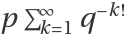for a rational number r=p/q.
The regular Liouville number corresponds to r=1/10.

## Examples

### Basic Examples (2)

Compute the Liouville number:

 In:=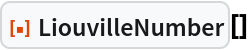Out=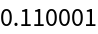Compute the Liouville number to 403 digits:

 In:=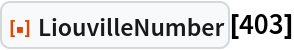Out=Compute the Liouville number corresponding to 14/33 to 1096 digits:

 In:=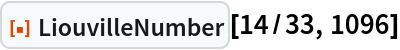Out=### Properties and Relations (2)

Large integers sporadically appear in the continued fraction expansion of the Liouville number:

 In:=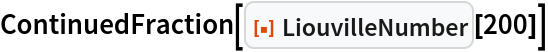Out=The Liouville number is very nearly equal to the root of a certain sixth-degree polynomial:

 In:=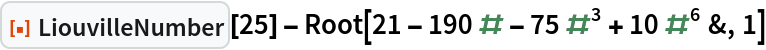Out=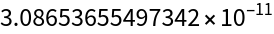### Neat Examples (1)

Plot values of the first few Liouville numbers corresponding to 1/b:

 In:=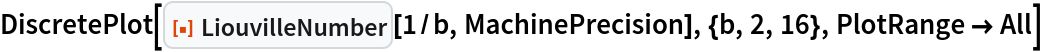Out=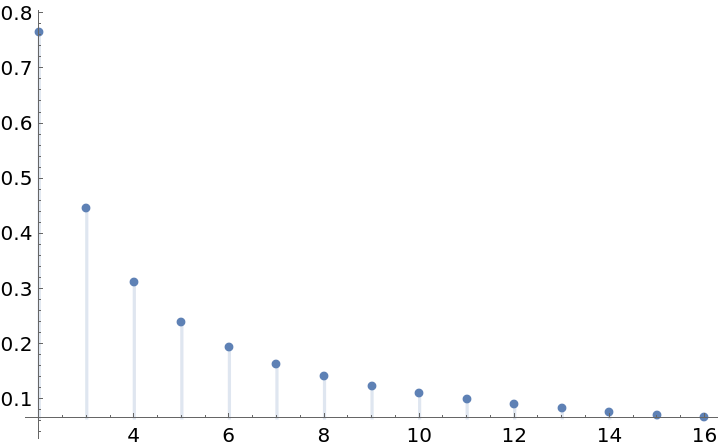Peter Burbery

## Version History

• 1.0.0 – 30 August 2022